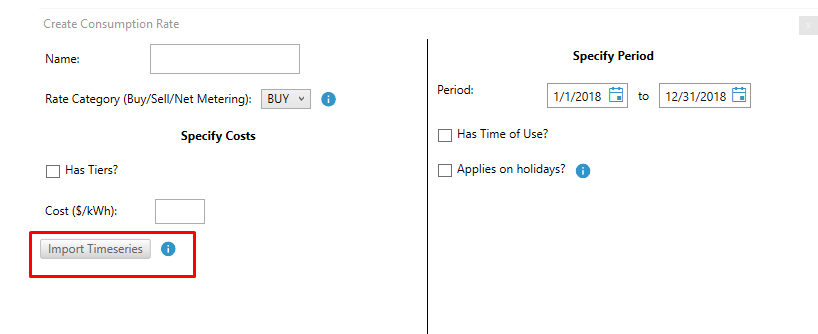HOMER Grid 1.8

 Consumption RateA consumption rate (\$/kWh) can be used to calculate a bill in different ways, depending on whether there is excess solar production and whether selling to the grid is allowed. Many utilities have designed complex meter structures to keep track of the purchases from grid and the sales to the grid. Feed-in tariffs, Net metering, Buy-all Sell-all are all different types of consumption rates and have different definitions around the world. To keep it simple, we use the following five rate transaction types to define how a consumption rate is used to calculate the bill. A tariff can have many consumption rates and each consumption rate can have only one rate transaction type. Here’s a list of the possible rate transaction types:

 Transaction Type How the bill is calculated Net Meter cost = Net kWh * \$/kWh Net Excess IF Net kWh<0, the cost applies= Net kWh * \$/kWh Net Purchase IF Net kWh>0, the cost applies= Net kWh * \$/kWh Import For the sum of instantaneous energy (kWh) coming from grid, apply cost= Purchases kWh * \$kWh Export For the sum of instantaneous energy (kWh) sent to grid, apply cost = (-) Sales kWh * \$/kWh

These transaction types are further explained below:

Understanding Net Meter, Net Excess, and Net Purchase:

The diagram below shows the transaction between solar panel, house (customer) and utility using a single meter.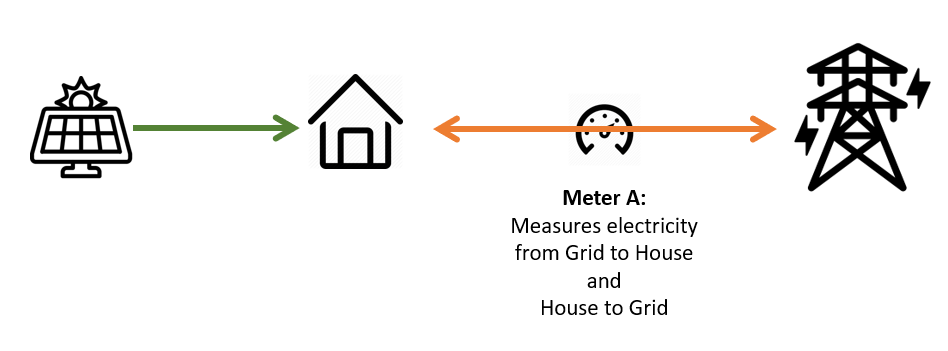The house has only one meter, which can “move forward” when buying power from the grid and “move backward” when selling excess to the grid.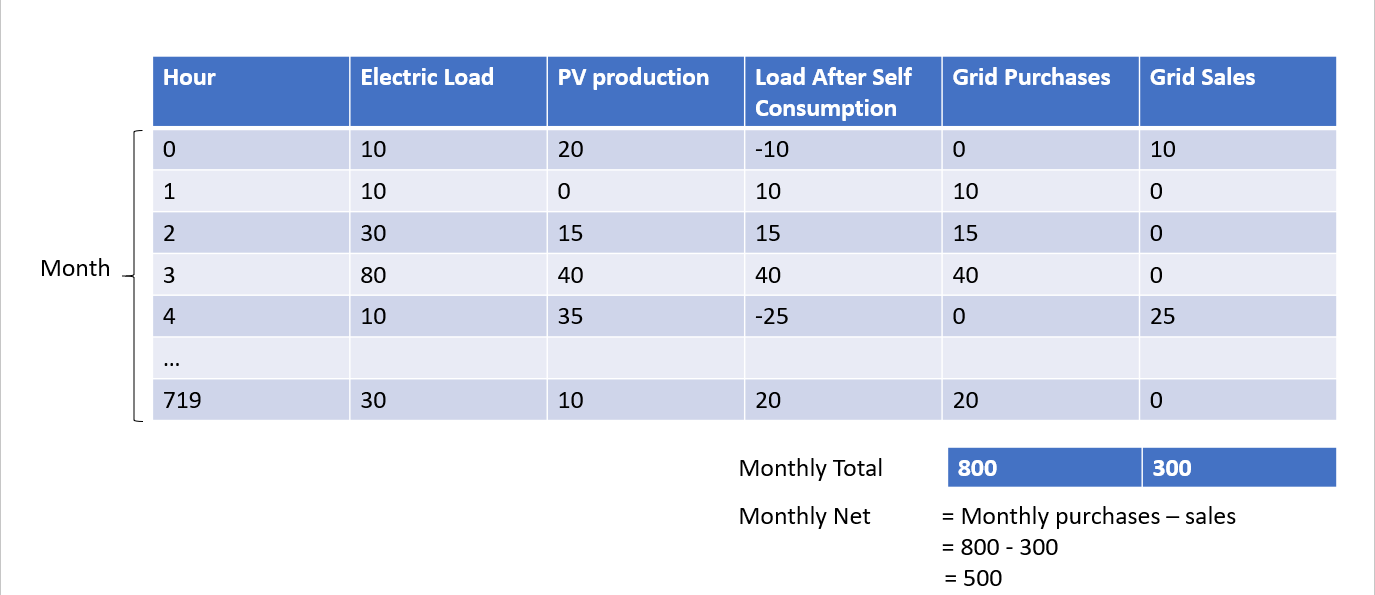For the first hour in the month of January, the load was 10 kW, and the solar panel produced 20 kW. In that hour, the load after self-consumption is -10 kW. Since it is a negative load, we could export energy to the grid. Therefore, in the first hour in January, the system sold 10 kW to the Grid. After the first hour in January, meter A now has “moved backwards” and has recorded -10.

In the next hour, the load after self-consumption is 10 kW, meaning the system needs to purchase 10 kW from the grid. Now the meter will move forward by ten and will read zero (-10 + 10 =0). This goes on until end of the month when the meter is read by the utility.

In this type of meter, only the monthly “net” reading affect the utility bill – not the instantaneous meter readings. If the total purchases at the end of the month have been greater than total sales, then the meter will have a positive value. If the total sales at the end of the month is greater than total purchases, then the meter will have a negative value.

To represent meters that record the “net” consumption over a period of time, we use the following three rate transaction types:

(1)Net Meter

A consumption rate with “NetMeter” will be calculated as = Net kWh * \$/kWh.

In the above example, the Net kWh were calculated over the period of a month. Some utilities will have different charge periods. The charge period options for “NetMeter” rates are: Hourly, Daily, Monthly, and Annually.

(2)Net Purchase

A consumption rate with “NetPurchase” will be calculated ONLY IF Net kWh>0. The cost = Net kWh * \$/kWh.

In the above example, the Net kWh were calculated over the period of a month. Some utilities will have different charge periods. The charge period options for “NetPurchase” rates are: Hourly and Monthly.

(3)Net Excess

A consumption rate with “NetExcess” will be calculated ONLY IF Net kWh<0. The cost = Net kWh * \$/kWh

In the above example, the Net kWh were calculated over the period of a month. Some utilities will have different charge periods. The charge options for “NetExcess” rates are: Hourly and Monthly.

Understanding Import and Export

The diagram below shows the energy flows between a solar array, a house (customer), and the utility.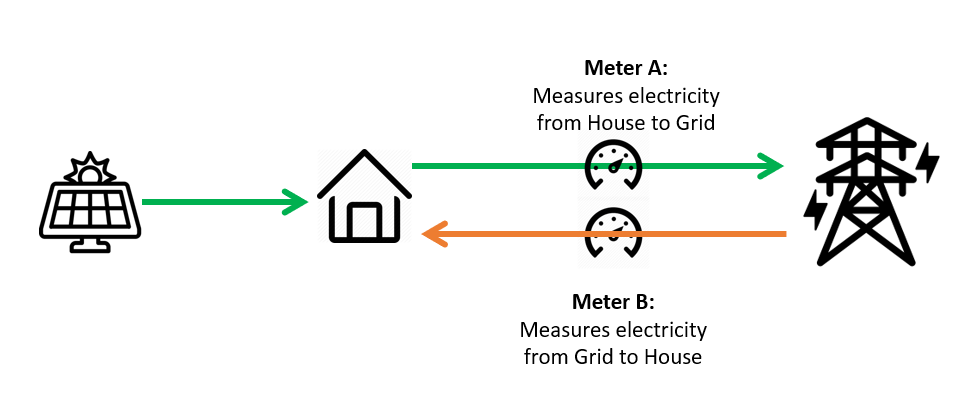The system has two meters, each of which can only “move forwards”.  Meter A will record any electricity sold to the grid. Meter B will record any electricity purchased from the grid. Another way to look at this is that Meter A and B will record instantaneous exports and imports, respectively. There is no “netting” at the end of the month or charge period.

To represent Meter A, we have:

(4)Export

A consumption rate with “Export” will be calculated as = (-) Sum of instantaneous Sales kWh * \$/kWh

To represent Meter B, we have:

(5)Import

A consumption rate with “Import” will be calculated as = Sum of instantaneous Purchases kWh * \$/kWh

The estimated cost for these two rate transaction types is calculated on a monthly basis. Comparing Transaction Types:

Below is an example of the different calculations by rate type with a monthly charge:

 \$/kWh 0.5 0.2 0.5 0.5 0.2 Month Energy Purchased Energy Sold Net Energy Net Purchase Net Excess Net Meter Import Export kWh kWh kWh \$ \$ \$ \$ \$ Jan 72,071 61,007 11,064 5,532 0 5,532 36,036 -12,201 Feb 59,490 57,856 1,634 817 0 817 29,745 -11,571 Mar 60,907 67,186 -6,278 0 -1,256 -3,139 30,454 -13,437 Apr 52,656 61,070 -8,414 0 -1,683 -4,207 26,328 -12,214 May 56,282 60,666 -4,384 0 -877 -2,192 28,141 -12,133 Jun 60,384 51,916 8,468 4,234 0 4,234 30,192 -10,383 Jul 62,993 52,762 10,231 5,116 0 5,116 31,497 -10,552 Aug 68,213 56,097 12,116 6,058 0 6,058 34,107 -11,219 Sep 62,066 63,651 -1,584 0 -317 -792 31,033 -12,730 Oct 61,313 64,410 -3,097 0 -619 -1,549 30,657 -12,882 Nov 71,771 52,437 19,334 9,667 0 9,667 35,886 -10,487 Dec 72,843 55,752 17,091 8,546 0 8,546 36,422 -11,150

The table shows that the estimated utility bill depends significantly on which transaction type is defined.

You can add multiple consumption or energy charges to your tariff. Click on the Add Rate button on the right corner under the consumption tab.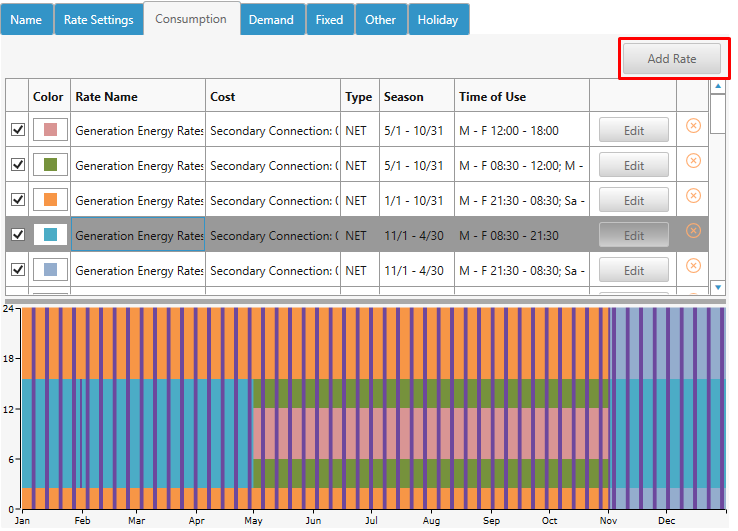This will open a pop-up window as shown below.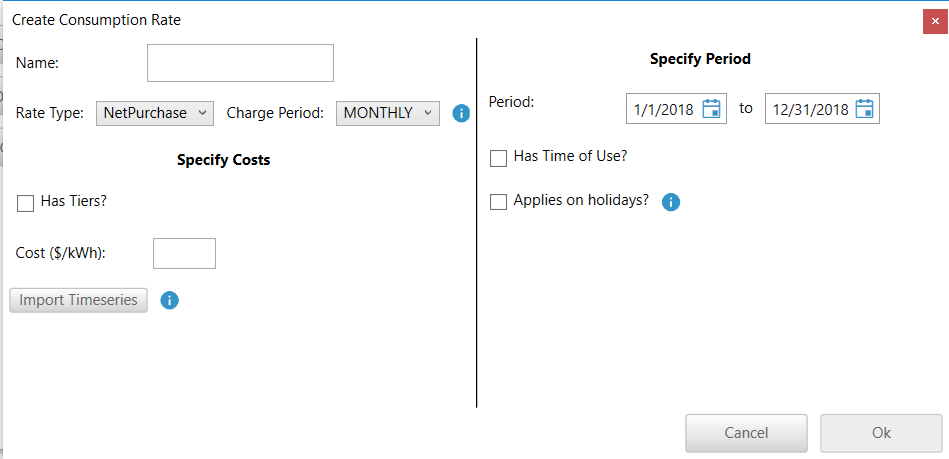Step 1: Enter the name of the consumption rate.Step 2: Select the rate type and the corresponding charge period.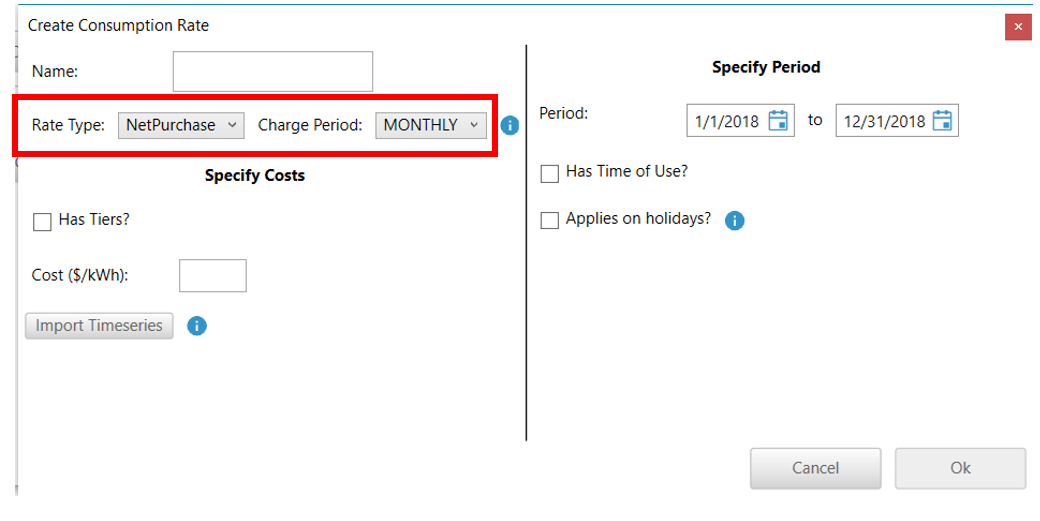As discussed in the beginning of this article the following charge periods are available for each rate type.

 Rate Type Charge Periods NetMeter Hourly, Daily, Monthly and Annually NetPurchase Hourly and Monthly NetExcess Hourly and Monthly Import Monthly Export Monthly

Step 3: Specify the cost

a. Flat energy rate - If it is a constant energy rate (\$/kWh) then enter the cost in box for Cost (\$/kWh)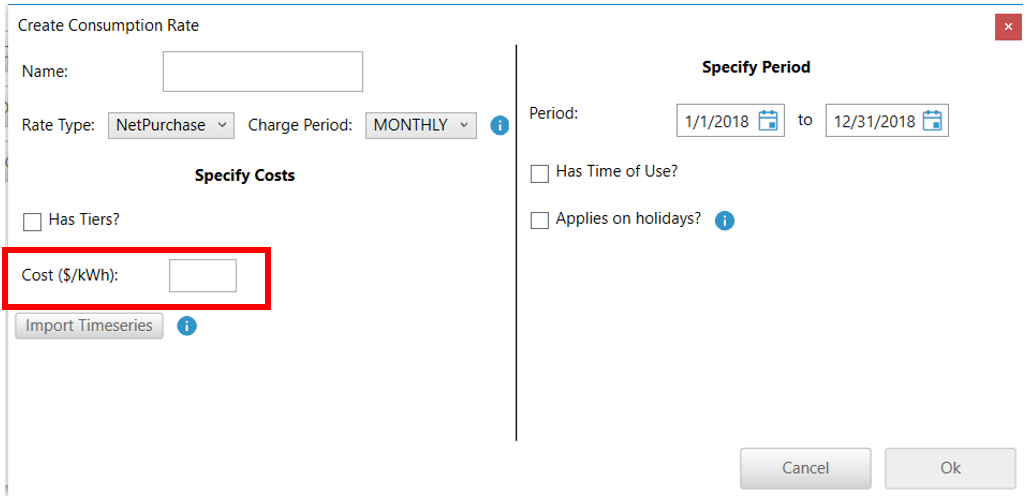b. Tiered energy rate- If it is a tiered energy rate, then check the box that asks “Has Tiers”. This displays a table for you to enter the tiers and their costs. Enter the Limits for each Tier and the costs in \$/kWh. The table in the picture below, has the following tiers - from 0 to 50 kWh, the rate is \$0.5 per kWh. Between 50 -100 kWh the rate is \$1 per kWh. Any consumption above 100 kWh would be charges \$2 per kWh.

So if a customer consumed 300 kWh in the billing period, then the cost = ( 50 kWh * 0.5 \$/kWh) + (100 kWh *1 \$/kWh) + (150 kWh * 2 \$/kWh) = \$425

Tip: Be sure to add a last tier with a really high upper limit so that all consumption that exceeds the previous limit will fall into this tier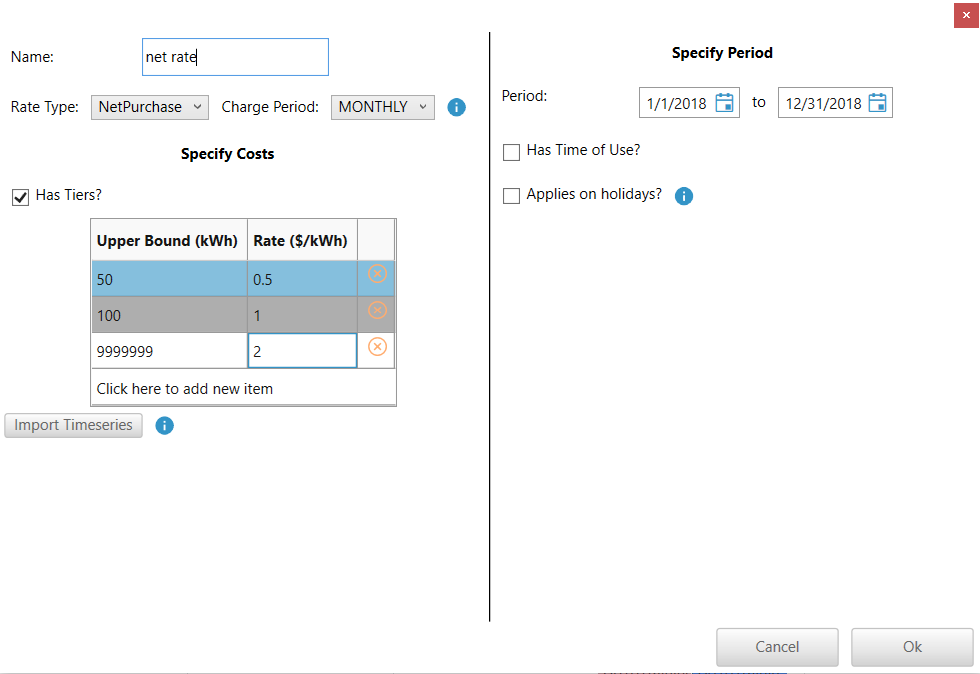Step 4: Specify the Period

a: If this rate applies to a certain time of year, change the start and end dates accordingly. The end date is included in the specified period.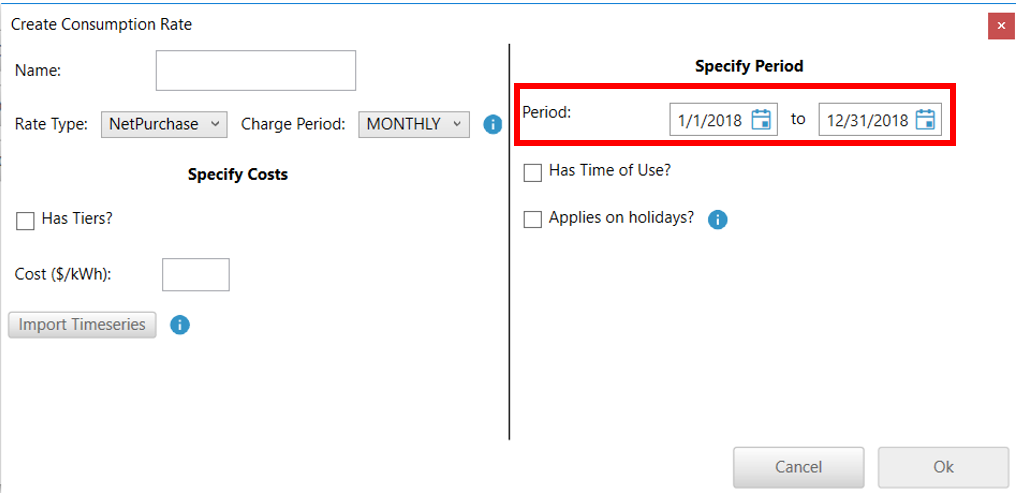b: If this rate has a Time of Use, check the box named “Has Time of Use?”.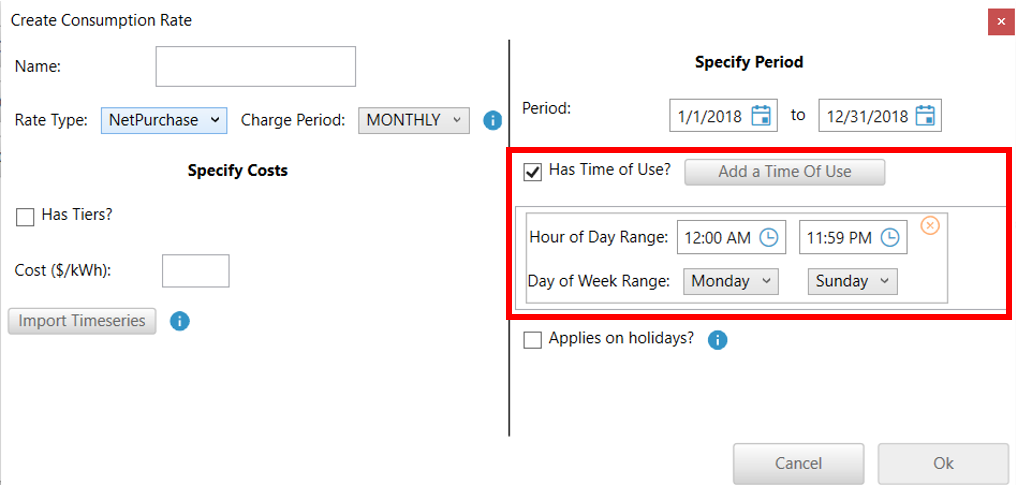Specify a time range and day of week range. You can specify multiple ranges by clicking the “Add a Time of Use” button as necessary.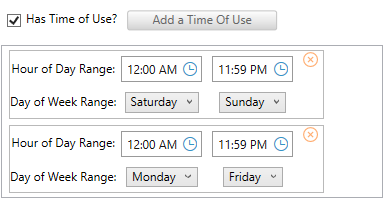Step 5: Specify if this rate applies during Holidays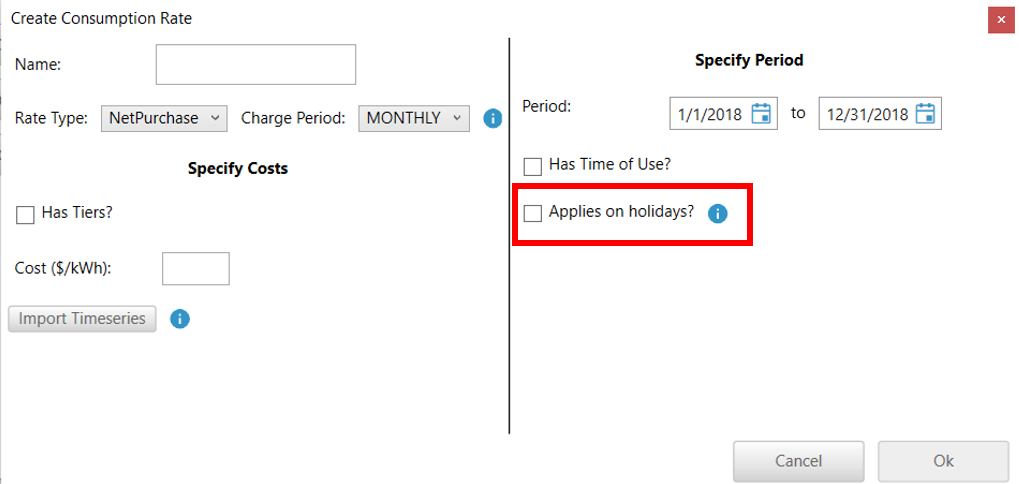If checked, this rate will apply during the entire day for each holiday, ignoring the Period and Time of Use. This box should be checked for only one consumption rate. If there is more than one consumption rate that includes holidays, only the last one will be applied to the holiday.